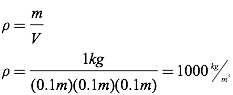# Density

Density is defined as the ratio of an object’s mass to the volume it occupies, and is frequently given the symbol rho (ρ) in physics.Less dense fluids will float on top of more dense fluids, and less dense solids will float on top of more dense fluids (keeping in mind you must look at the average density of the entire solid object).

Question: A single kilogram of water fills a cube of length 0.1m. What is the density of water?

Answer:Question: Gold has a density of 19,320 kg/m3. How much volume does a single kilogram of gold occupy?Answer:Question: Fresh water has a density of 1000 kg/m3. Which of the following materials will float on water?

1. Ice (ρ=917 kg/m3)
2. Magnesium (ρ=1740 kg/m3)
3. Cork (ρ=250 kg/m3)
4. Glycerol (ρ=1261 kg/m3)

Answer: Both ice and cork will float on water because they have an average density less than that of water.

Question: Based on the image below, what can you say about the average density of the man and inner tube compared to the density of the water?

1. The average density of the man and inner tube is greater than that of the water.
2. The average density of the man and inner tube is less than that of the water.
3. The average density of the man and inner tube is equal to that of the water.Answer: (2) The average density of a solid must be less than that of any fluid it is floating in.